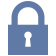Updated: 9/14/2019

# Statistical Distributions

0%
Topic
Review Topic
0
0
N/A
N/A
Questions
7
0
0
Topic
• Descriptive Statistics
• Mean
• Average of all observation
• Mean = (sum of all observations)/(sample size)
• Median
• The middle value of all observations
• If sample size is odd
• Median = ((n+1)/2)th largest value
• If the sample size is even
• Median = the average of the (n/2)th and ((n/2)+1)th largest value
• Mode
• The most commonly occurring value
• If there is more than one most commonly occurring value, there are as many modes as most commonly occurring values
• Types of Distributions
• Normal
• aka Gaussian, bell-shaped
• Mean = median = mode
• Bi-modal
• Distribution has two humps (each being a relative mode)
• If symmetrical, mean = median
• Skewed
• Positive Skew
• Asymmetrical with tail trailing off to right
• Mean > median > model
• Negative Skew
• Asymmetrical with tail trailing off to left
• Mean < median < mode
• Mean very sensitive to skew
• Median somewhat resistant to skew
• Mode very resistant to skew
• Characteristics of the Normal Distribution
• Defined entirely by two parameters
• Mean (µ)
• Standard deviation (σ)
• A certain percentage of all observations will always fall within +/- certain standard deviations of the mean
• +/- 1 Standard deviation = 68%
• +/- 2 Standard deviations = 95%
• +/- 3 Standard deviations = 99.7%

You have never rated this topic.

Thank you. You can rate this topic again in 12 months.

Flashcards (0)
Cards
1 of 0
Questions (7)

(M2.OMB.18.1) A study on cholesterol levels is performed. There are 1000 participants. It is determined that in this population, the mean LDL is 200 mg/dL with a standard deviation of 50 mg/dL. If the population has a normal distribution, how many people have a cholesterol less than 300 mg/dL?

QID: 210444

680

8%

(1/12)

840

0%

(0/12)

950

17%

(2/12)

975

75%

(9/12)

997

0%

(0/12)

M 5 B

Select Answer to see Preferred Response

(M2.OMB.18.1) A patient is in the ICU for diabetic ketoacidosis and is currently on an insulin drip. His electrolytes are being checked every hour and his potassium is notable for the following measures:

1. 5.1 mEq/L
2. 5.8 mEq/L
3. 6.1 mEq/L
4. 6.2 mEq/L
5. 5.9 mEq/L
6. 5.1 mEq/L
7. 4.0 mEq/L
8. 3.1 mEq/L

Which of the following is the median potassium value of this data set?

QID: 210439

3.10

14%

(2/14)

5.10

21%

(3/14)

5.16

21%

(3/14)

5.45

36%

(5/14)

6.05

0%

(0/14)

M 5 C

Select Answer to see Preferred ResponseSorry, this question is forSorry, this question is for